# UVIS Time-dependent photometry¶

### Description¶

#### This jupyter notebook demonstrates how to apply the new time-dependent inverse sensitivities (zeropoints) using the PHOTFLAM header keyword to sample data.¶

The notebook comes with example standard star (GD153) flat-fielded CTE-corrected (FLC) files for three separate epochs in the filter F606W and C512C subarray mode. It also comes with a CSV file that contains a list of FLCs, and the centroids of the star for each FLC image.

## Imports¶

In :
import glob
import matplotlib.pyplot as plt
import numpy as np
import pandas as pd

from astropy.io import fits
from astropy.stats import sigma_clipped_stats
from astropy.coordinates import SkyCoord
from astropy import wcs

from drizzlepac import photeq
from drizzlepac import astrodrizzle

from photutils import aperture_photometry, CircularAperture, CircularAnnulus

from stwcs import updatewcs

%matplotlib inline

The following task in the stsci.skypac package can be run with TEAL:
skymatch
The following tasks in the drizzlepac package can be run with TEAL:
astrodrizzle       config_testbed      imagefindpars           mapreg
photeq            pixreplace           pixtopix            pixtosky
refimagefindpars       resetbits          runastrodriz          skytopix
tweakback            tweakreg           updatenpol

In :
df = pd.read_csv('GD153_F606W_public.csv')

In :
df

Out:
FLC Centx Centy Filter Amp Chip Epoch
0 ibcda4d0q_flc.fits 289.940 285.934 F606W C 2 1
1 ibcda4d6q_flc.fits 325.855 299.742 F606W C 2 1
2 ibcda4dcq_flc.fits 312.830 321.911 F606W C 2 1
3 ibcda4diq_flc.fits 276.756 308.288 F606W C 2 1
4 ich304prq_flc.fits 246.181 331.752 F606W C 2 2
5 ich304q1q_flc.fits 248.901 334.022 F606W C 2 2
6 idbha6ntq_flc.fits 262.719 254.764 F606W C 2 3
7 idbha6nyq_flc.fits 265.020 257.049 F606W C 2 3

### Pixel Area Maps (PAM)¶

FLC frames are not corrected for distortion and pixels therefore do not have equal area on the sky. To correct for this affect, we multiply the FLC frames by the Pixel Area Map. If you're using full-frame UVIS images, you do not need the dictionary below. Since the GD153 data we're looking at here are C512 subarrays, the PAM needs to be "cut out" at the region corresponding to the subarray.

In :
pam1 = fits.getdata('UVIS1wfc3_map.fits')
pam2 = fits.getdata('UVIS2wfc3_map.fits')

pams = {'A': pam1[-512:, :513], 'B': pam1[-512:, -513:], 'C': pam2[:512, :513], 'D': pam2[:512, -513:]}


### Photometry with no correction¶

Here we set up the code that computes the photometry. We are using a standard aperture size of 10 pixels, with a sky annulus from 155 to 165 pixels. These are the steps involved in computing the photometry:

1. Loop through each FLC and load the data, MJD date of exposure, exposure time, and PHOTFLAM
2. Divide the data by the exposure time to get pixel values in electrons per second (as opposed to just electrons)
3. Multiply this result by the PAM, or the cutout of the PAM corresponding to the specific region of the subarray
4. Supply the centroid of the object from the CSV file/pandas dataframe
5. Set up the aperture, annulus aperture, and annulus mask
6. Compute the sigma-clipped mean of the annulus
7. Compute the photometry in the aperture
8. Subtract the background measurement (derived in step 6) from the photometry derived in step 7.
9. Store all values
In :
phots = []
mjds = []
ap = 10
pfl = []
for i, flc in enumerate(df['FLC'].values):
with fits.open(flc) as f:
data = f.data
data = data / exptime
data = data * pams[df.at[i, 'Amp']]

positions = (df.at[i, 'Centx'], df.at[i, 'Centy'])
aperture = CircularAperture(positions, ap)
mean_sigclip, _, _ = sigma_clipped_stats(annulus_data_1d)
background = mean_sigclip * aperture.area

apers = [aperture, annulus_aperture]
phot_table = aperture_photometry(data,apers)

final_sum = phot_table['aperture_sum_0'] - background
phots.append(final_sum)
mjds.append(mjd)

df['Countrate'] = phots
df['MJD'] = mjds
df['PHOTFLAM'] = pfl


### Magnitudes¶

We can convert countrates into ST magnitudes using the zeropoint indicated in PHOTFLAM, and using the following equation. The EE_r10 is the encircled energy term for an aperture radius of r=10 pixels (0.4 arcseconds). For F606W, this is 0.91. This value can be computed using pysynphot.

In :
EE_r10 = 0.91
df['STMags'] = -21.1 -2.5*np.log10(df['PHOTFLAM']) -2.5*np.log10(df['Countrate']) - 2.5 * np.log10(1./EE_r10)

In :
df

Out:
FLC Centx Centy Filter Amp Chip Epoch Countrate MJD PHOTFLAM STMags
0 ibcda4d0q_flc.fits 289.940 285.934 F606W C 2 1 102800.696778 55170.563332 1.164713e-19 13.602066
1 ibcda4d6q_flc.fits 325.855 299.742 F606W C 2 1 102793.059557 55170.569026 1.164713e-19 13.602146
2 ibcda4dcq_flc.fits 312.830 321.911 F606W C 2 1 103160.884751 55170.574721 1.164713e-19 13.598268
3 ibcda4diq_flc.fits 276.756 308.288 F606W C 2 1 102950.002844 55170.620196 1.164714e-19 13.600489
4 ich304prq_flc.fits 246.181 331.752 F606W C 2 2 101958.945608 56629.866744 1.174709e-19 13.601715
5 ich304q1q_flc.fits 248.901 334.022 F606W C 2 2 102227.291086 56629.877033 1.174709e-19 13.598861
6 idbha6ntq_flc.fits 262.719 254.764 F606W C 2 3 101316.075091 58068.856082 1.184584e-19 13.599493
7 idbha6nyq_flc.fits 265.020 257.049 F606W C 2 3 101047.930892 58068.861359 1.184584e-19 13.602370

## Plotting Countrates¶

We first plot the photometric countrates (electrons per second) over time in MJD. Note that you can see the decline in the countrates over time, due to the expected and documented sensitivity losses for the instrument

In :
fig = plt.figure(figsize=(40, 25), dpi=40)

plt.plot(df['MJD'], df['Countrate'], 'o', markersize=40, label='Amp C')
plt.grid()
plt.xlabel('MJD', fontsize=40)
plt.xticks(fontsize=40)
plt.yticks(fontsize=40)
plt.ylabel('Countrate (e-/s)', fontsize=40)
plt.title('GD153, F606W, UVIS2', fontsize=40)
plt.ylim(101000, 104000)
plt.legend(loc=0, fontsize=40)

Out:
<matplotlib.legend.Legend at 0x7fdce9568c18>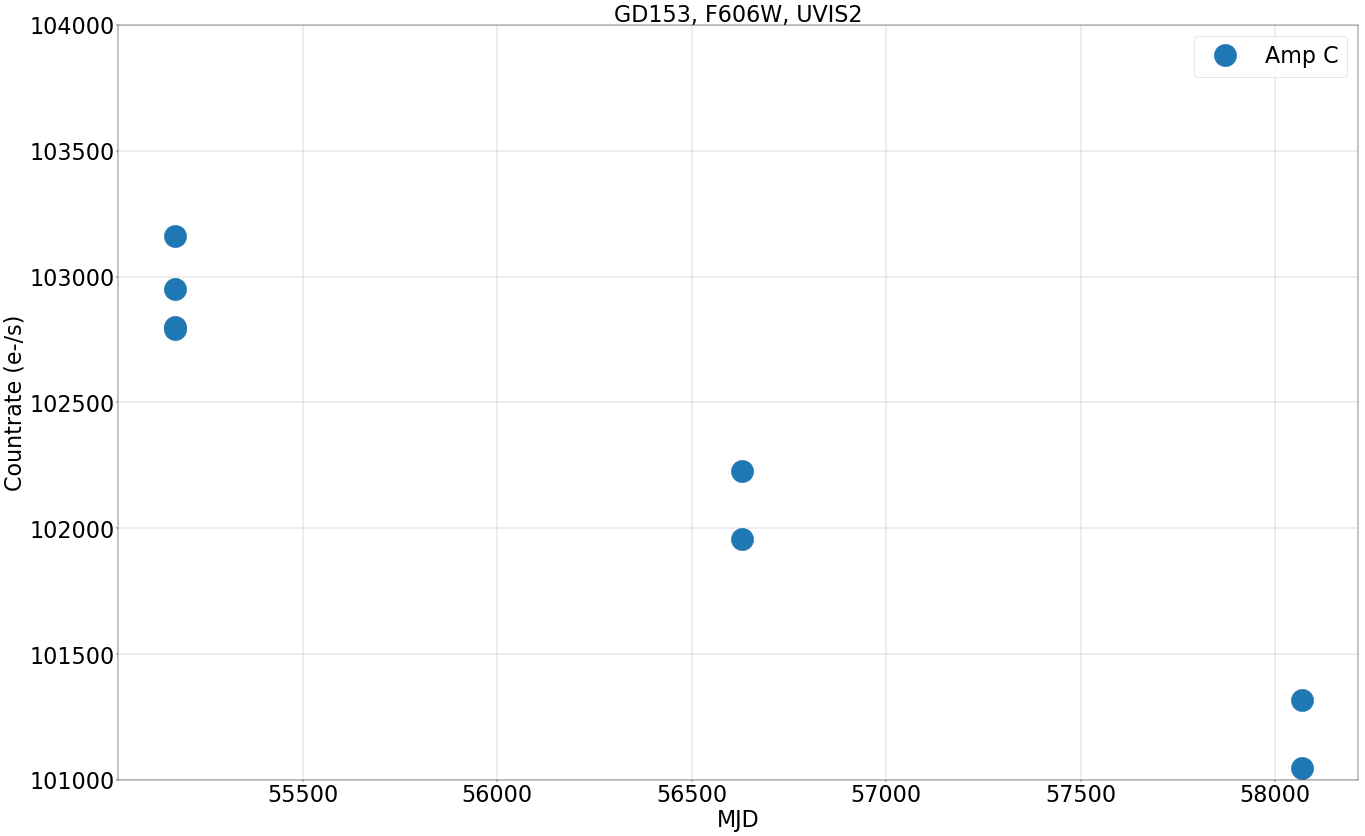## Plotting Magnitudes¶

Now we plot the ST magnitude over time in MJD. This is using the corrected PHOTFLAM keyword, so as you can see the magnitudes are stable over time.

In :
fig = plt.figure(figsize=(40, 25), dpi=40)

plt.plot(df['MJD'], df['STMags'], 'o', markersize=40, label='Amp C')
plt.grid()
plt.ticklabel_format(useOffset=False)
plt.xlabel('MJD', fontsize=40)
plt.xticks(fontsize=40)
plt.yticks(fontsize=40)
plt.ylabel('STMAG (Magnitude)', fontsize=40)
plt.ylim(13.55, 13.65)
plt.title('GD153, F606W, UVIS2', fontsize=40)
plt.legend(loc=0, fontsize=40)

Out:
<matplotlib.legend.Legend at 0x7fdcd10329e8>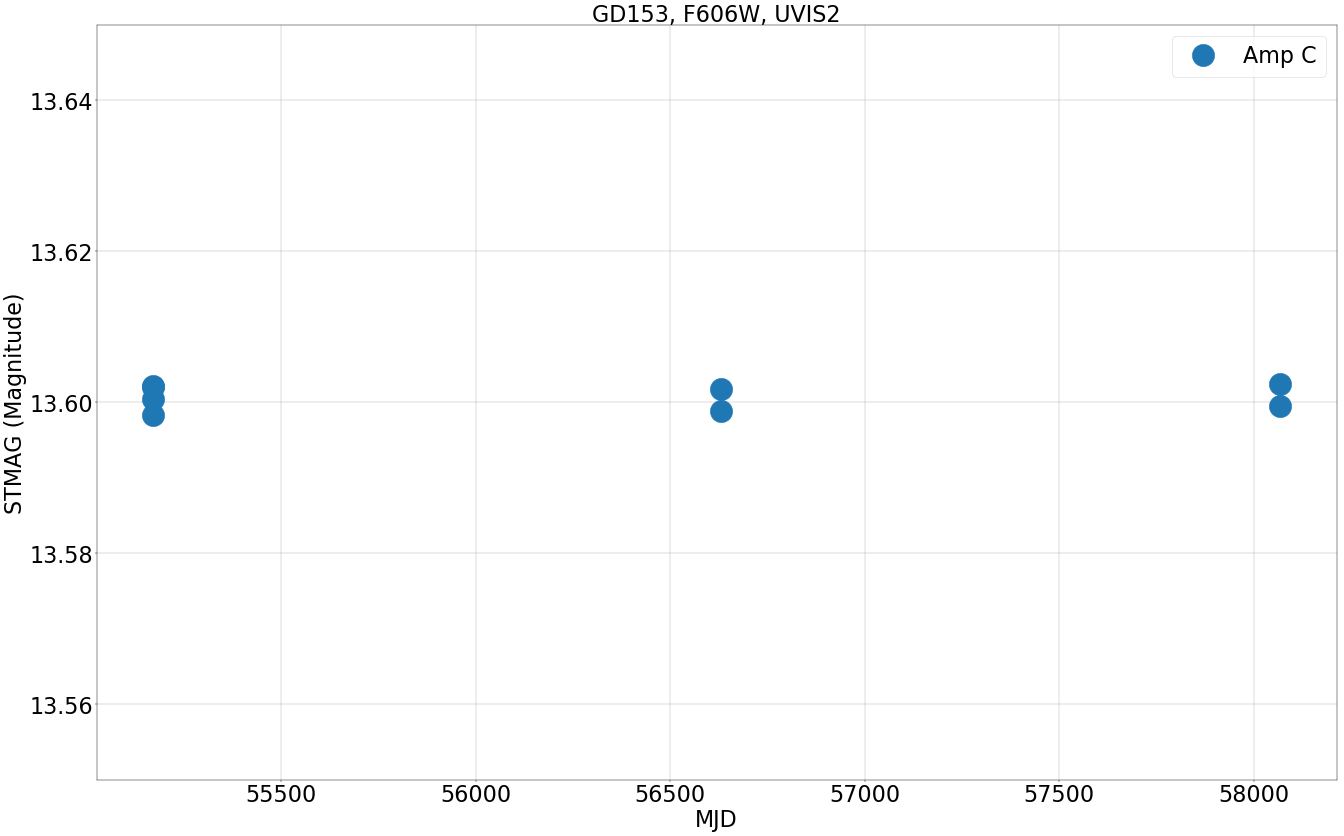### Photometric equalization¶

This simple single-line step will equalize the countrates in the science array of the FLC frames to match any specified 'reference' image. For more details, see the phot_eq software documentation. Note that at this step we overwrite the science pixels in the original FLC files. Note that in this case the data are sorted, and the step automatically uses the 2009 GD153 data PHOTFLAM values to match the rest of the images to. You can supply a given reference PHOTFLAM value to the photeq call or/and ensure that your data are time-sorted

In :
photeq.photeq(','.join(df['FLC'].values), readonly=False)

***** drizzlepac.photeq started on 2020-10-28 16:05:50.360047
Version 0.2 (06-Nov-2015)

PRIMARY PHOTOMETRIC KEYWORD: PHOTFLAM
SECONDARY PHOTOMETRIC KEYWORD(S): PHOTFNU
REFERENCE VALUE FROM FILE: 'ibcda4d0q_flc.fits'
REFERENCE 'PHOTFLAM' VALUE IS: 1.1647135e-19

Processing file 'ibcda4d0q_flc.fits'
- 'PHOTFLAM' = 1.1647135e-19 found in the primary header.
- Data conversion factor based on primary header: 1.0
* EXT: ('SCI', 1)
- Setting PHOTFLAM to 1.1647135e-19 (old value was 1.1647135e-19)
- Computed conversion factor for data: 1.0
- Setting PHOTFNU to 1.3501655e-07 (old value was 1.3501655e-07)
- Data have been multiplied by 1.0
- Error array (ext=('ERR', 1)) has been multiplied by 1.0

Processing file 'ibcda4d6q_flc.fits'
- 'PHOTFLAM' found in the primary header.
- Setting PHOTFLAM in the primary header to 1.1647135e-19 (old value was 1.1647135e-19)
- Data conversion factor based on primary header: 1.0
* EXT: ('SCI', 1)
- Setting PHOTFLAM to 1.1647135e-19 (old value was 1.1647135e-19)
- Computed conversion factor for data: 1.0
- Setting PHOTFNU to 1.3501655e-07 (old value was 1.3501655e-07)
- Data have been multiplied by 1.0
- Error array (ext=('ERR', 1)) has been multiplied by 1.0

Processing file 'ibcda4dcq_flc.fits'
- 'PHOTFLAM' found in the primary header.
- Setting PHOTFLAM in the primary header to 1.1647135e-19 (old value was 1.1647135e-19)
- Data conversion factor based on primary header: 1.0
* EXT: ('SCI', 1)
- Setting PHOTFLAM to 1.1647135e-19 (old value was 1.1647135e-19)
- Computed conversion factor for data: 1.0
- Setting PHOTFNU to 1.3501655e-07 (old value was 1.3501655e-07)
- Data have been multiplied by 1.0
- Error array (ext=('ERR', 1)) has been multiplied by 1.0

Processing file 'ibcda4diq_flc.fits'
- 'PHOTFLAM' found in the primary header.
- Setting PHOTFLAM in the primary header to 1.1647135e-19 (old value was 1.1647139e-19)
- Data conversion factor based on primary header: 1.0000003434320972
* EXT: ('SCI', 1)
- Setting PHOTFLAM to 1.1647135e-19 (old value was 1.1647139e-19)
- Computed conversion factor for data: 1.0000003434320972
- Setting PHOTFNU to 1.350165336309887e-07 (old value was 1.3501658e-07)
- Data have been multiplied by 1.0000003434320972
- Error array (ext=('ERR', 1)) has been multiplied by 1.0000003434320972

Processing file 'ich304prq_flc.fits'
- 'PHOTFLAM' found in the primary header.
- Setting PHOTFLAM in the primary header to 1.1647135e-19 (old value was 1.1747087e-19)
- Data conversion factor based on primary header: 1.0085816812460746
* EXT: ('SCI', 1)
- Setting PHOTFLAM to 1.1647135e-19 (old value was 1.1747087e-19)
- Computed conversion factor for data: 1.0085816812460746
- Setting PHOTFNU to 1.3478350095755228e-07 (old value was 1.3594017e-07)
- Data have been multiplied by 1.0085816812460746
- Error array (ext=('ERR', 1)) has been multiplied by 1.0085816812460746

Processing file 'ich304q1q_flc.fits'
- 'PHOTFLAM' found in the primary header.
- Setting PHOTFLAM in the primary header to 1.1647135e-19 (old value was 1.1747088e-19)
- Data conversion factor based on primary header: 1.008581767104099
* EXT: ('SCI', 1)
- Setting PHOTFLAM to 1.1647135e-19 (old value was 1.1747088e-19)
- Computed conversion factor for data: 1.008581767104099
- Setting PHOTFNU to 1.3478349939868502e-07 (old value was 1.3594018e-07)
- Data have been multiplied by 1.008581767104099
- Error array (ext=('ERR', 1)) has been multiplied by 1.008581767104099

Processing file 'idbha6ntq_flc.fits'
- 'PHOTFLAM' found in the primary header.
- Setting PHOTFLAM in the primary header to 1.1647135e-19 (old value was 1.184584e-19)
- Data conversion factor based on primary header: 1.0170604187209988
* EXT: ('SCI', 1)
- Setting PHOTFLAM to 1.1647135e-19 (old value was 1.184584e-19)
- Computed conversion factor for data: 1.0170604187209988
- Setting PHOTFNU to 1.345667351523826e-07 (old value was 1.368625e-07)
- Data have been multiplied by 1.0170604187209988
- Error array (ext=('ERR', 1)) has been multiplied by 1.0170604187209988

Processing file 'idbha6nyq_flc.fits'
- 'PHOTFLAM' found in the primary header.
- Setting PHOTFLAM in the primary header to 1.1647135e-19 (old value was 1.1845842e-19)
- Data conversion factor based on primary header: 1.0170605904370476
* EXT: ('SCI', 1)
- Setting PHOTFLAM to 1.1647135e-19 (old value was 1.1845842e-19)
- Computed conversion factor for data: 1.0170605904370476
- Setting PHOTFNU to 1.3456671243272534e-07 (old value was 1.368625e-07)
- Data have been multiplied by 1.0170605904370476
- Error array (ext=('ERR', 1)) has been multiplied by 1.0170605904370476

Done.


### Repeating photometric Countrate calculation¶

We repeat the same computation as earlier for the photometric Countrate, but using the now photometrically equalized data.

In :
phots = []
for i, flc in enumerate(df['FLC'].values):
with fits.open(flc) as f:
data = f.data
data = data / exptime
data = data * pams[df.at[i, 'Amp']]

positions = (df.at[i, 'Centx'], df.at[i, 'Centy'])
aperture = CircularAperture(positions, ap)
mean_sigclip, _, _ = sigma_clipped_stats(annulus_data_1d)
background = mean_sigclip * aperture.area

apers = [aperture, annulus_aperture]
phot_table = aperture_photometry(data,apers)

final_sum = phot_table['aperture_sum_0'] - background
phots.append(final_sum)
mjds.append(mjd)

df['Phot-eq'] = phots

In :
df

Out:
FLC Centx Centy Filter Amp Chip Epoch Countrate MJD PHOTFLAM STMags Phot-eq
0 ibcda4d0q_flc.fits 289.940 285.934 F606W C 2 1 102800.696778 55170.563332 1.164713e-19 13.602066 102800.696778
1 ibcda4d6q_flc.fits 325.855 299.742 F606W C 2 1 102793.059557 55170.569026 1.164713e-19 13.602146 102793.059557
2 ibcda4dcq_flc.fits 312.830 321.911 F606W C 2 1 103160.884751 55170.574721 1.164713e-19 13.598268 103160.884751
3 ibcda4diq_flc.fits 276.756 308.288 F606W C 2 1 102950.002844 55170.620196 1.164714e-19 13.600489 102950.039862
4 ich304prq_flc.fits 246.181 331.752 F606W C 2 2 101958.945608 56629.866744 1.174709e-19 13.601715 102833.918947
5 ich304q1q_flc.fits 248.901 334.022 F606W C 2 2 102227.291086 56629.877033 1.174709e-19 13.598861 103104.581990
6 idbha6ntq_flc.fits 262.719 254.764 F606W C 2 3 101316.075091 58068.856082 1.184584e-19 13.599493 103044.568745
7 idbha6nyq_flc.fits 265.020 257.049 F606W C 2 3 101047.930892 58068.861359 1.184584e-19 13.602370 102771.875076

## Plotting corrected Countrates¶

We plot the photometric Countrate (electrons per second) over time in MJD. You can now see that the data are much less variant over time. This is the corrected data

In :
fig = plt.figure(figsize=(40, 25), dpi=40)

plt.plot(df['MJD'], df['Countrate'], 's', markersize=50, label='Not equalized', alpha=0.55, color='Grey')
plt.plot(df['MJD'], df['Phot-eq'], 'o', markersize=40, label='Equalized')
plt.grid()
plt.xlabel('MJD', fontsize=40)
plt.xticks(fontsize=40)
plt.yticks(fontsize=40)
plt.ylabel('Countrate (e-/s)', fontsize=40)
plt.title('GD153, F606W, UVIS2 - Photometrically equalized', fontsize=40)
plt.ylim(101000, 104000)
plt.legend(loc=0, fontsize=40)

Out:
<matplotlib.legend.Legend at 0x7fdd08cff470>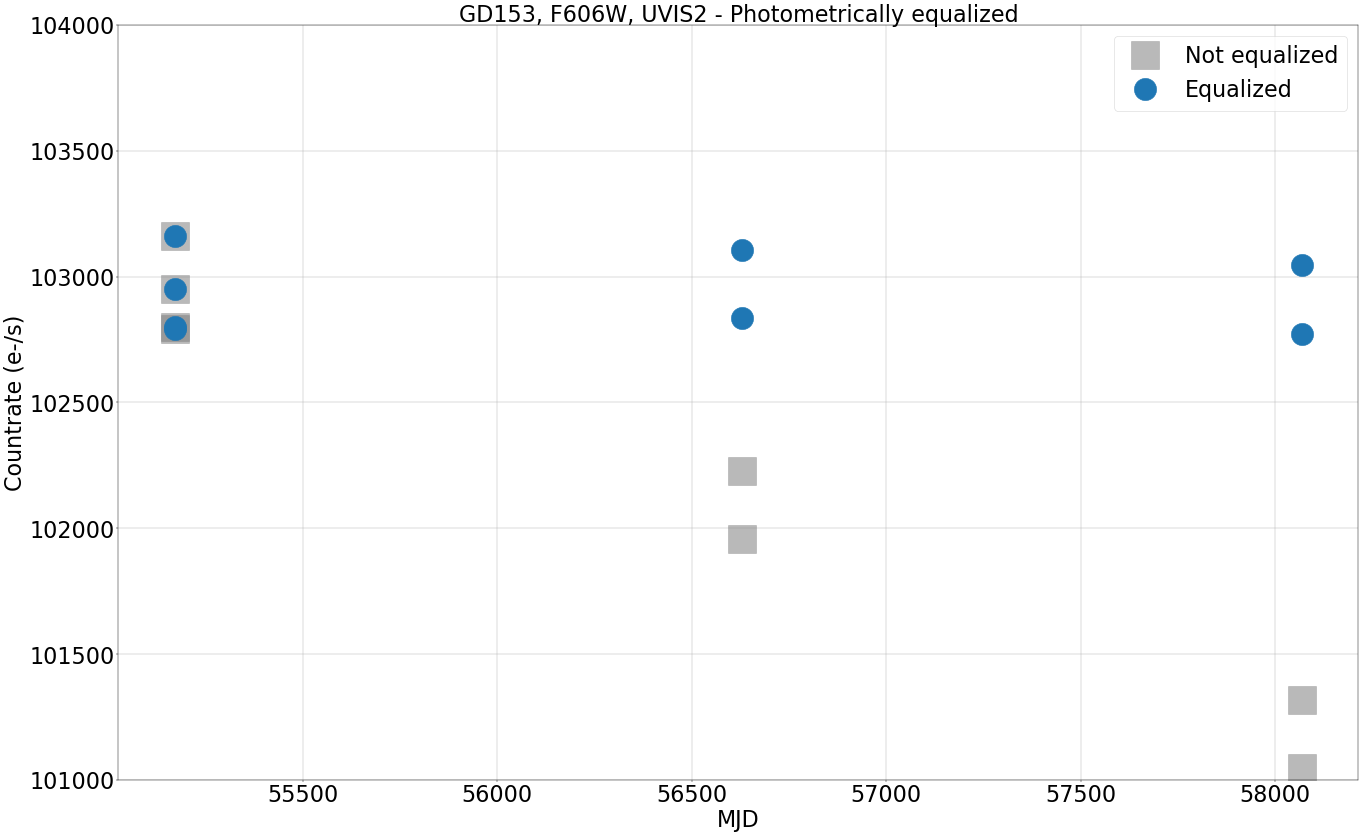## Performing Astrodrizzle and repeating photometry¶

The photometrically corrected data can be drizzled together (on a visit/epoch level basis) to correct for the effects of cosmic rays and to improve the signal-to-noise ratio.

We then perform the photometry on the finalized drizzle (DRC) products, using similar techniques as before. Drizzled data are in units of electrons per second and have the PAM already applied, so we no longer have to perform those two steps during photometry.

In :
for i in range(max(df['Epoch'])):
flcs = list(df.query('Epoch == {}'.format(i+1))['FLC'].values)
astrodrizzle.AstroDrizzle(flcs,
output= 'allCframes_{}'.format(flcs.split('_flc.fits')),
skymethod= 'match',
skystat='mean',
driz_sep_bits='80',
combine_type='median',
combine_nhigh=1,
driz_cr_snr= '3.5 3.0',
driz_cr_scale= '2.0 1.5',
final_bits= '80',
build=True,
clean=True,
preserve=False)

Setting up logfile :  astrodrizzle.log
AstroDrizzle Version 3.1.6 (2020-02-19 16:16:30 -0500) started at: 16:05:52.220 (28/10/2020)

==== Processing Step  Initialization  started at  16:05:52.25 (28/10/2020)

WCS Keywords

Number of WCS axes: 2
CTYPE : 'RA---TAN'  'DEC--TAN'
CRVAL : 194.258896779347  22.031359382276193
CRPIX : 282.5  290.5
CD1_1 CD1_2  : 1.0823471171761192e-05  1.9936712067124263e-06
CD2_1 CD2_2  : 1.9936712067124263e-06  -1.0823471171761192e-05
NAXIS : 565  581
********************************************************************************
*
*  Estimated memory usage:  up to 23 Mb.
*  Output image size:       565 X 581 pixels.
*  Output image file:       ~ 3 Mb.
*  Cores available:         4
*
********************************************************************************
==== Processing Step Initialization finished at 16:05:53.422 (28/10/2020)
==== Processing Step  Static Mask  started at  16:05:53.493 (28/10/2020)

==== Processing Step Static Mask finished at 16:05:53.634 (28/10/2020)
==== Processing Step  Subtract Sky  started at  16:05:53.639 (28/10/2020)

***** skymatch started on 2020-10-28 16:05:53.886149
Version 1.0.2 (2019-03-07 00:54:44 -0500)

'skymatch' task will apply computed sky differences to input image file(s).

NOTE: Computed sky values WILL NOT be subtracted from image data ('subtractsky'=False).
'MDRIZSKY' header keyword will represent sky value *computed* from data.

-----  User specified keywords:  -----
Sky Value Keyword:  'MDRIZSKY'
Data Units Keyword: 'BUNIT'

-----  Input file list:  -----

**  Input image: 'ibcda4d0q_flc.fits'

**  Input image: 'ibcda4d6q_flc.fits'

**  Input image: 'ibcda4dcq_flc.fits'

**  Input image: 'ibcda4diq_flc.fits'

-----  Sky statistics parameters:  -----
statistics function: 'mean'
lower = None
upper = None
nclip = 5
lsigma = 4.0
usigma = 4.0
binwidth = 0.1

-----  Data->Brightness conversion parameters for input files:  -----

*   Image: ibcda4d0q_flc.fits
EXT = 'SCI',1
Data units type: COUNTS
EXPTIME: 1.0 [s]
Conversion factor (data->brightness):  637.0463879342394

*   Image: ibcda4d6q_flc.fits
EXT = 'SCI',1
Data units type: COUNTS
EXPTIME: 1.0 [s]
Conversion factor (data->brightness):  637.0463879342394

*   Image: ibcda4dcq_flc.fits
EXT = 'SCI',1
Data units type: COUNTS
EXPTIME: 1.0 [s]
Conversion factor (data->brightness):  637.0463879342394

*   Image: ibcda4diq_flc.fits
EXT = 'SCI',1
Data units type: COUNTS
EXPTIME: 1.0 [s]
Conversion factor (data->brightness):  637.0463879342394

-----  Computing differences in sky values in overlapping regions:  -----

*   Image 'ibcda4d0q_flc.fits['SCI',1]' SKY = 69.514 [brightness units]
Updating sky of image extension(s) [data units]:
-  EXT = 'SCI',1   delta(MDRIZSKY) = 0.109119

*   Image 'ibcda4d6q_flc.fits['SCI',1]' SKY = 12.3352 [brightness units]
Updating sky of image extension(s) [data units]:
-  EXT = 'SCI',1   delta(MDRIZSKY) = 0.0193631

*   Image 'ibcda4dcq_flc.fits['SCI',1]' SKY = 13.9726 [brightness units]
Updating sky of image extension(s) [data units]:
-  EXT = 'SCI',1   delta(MDRIZSKY) = 0.0219334

*   Image 'ibcda4diq_flc.fits['SCI',1]' SKY = 0 [brightness units]
Updating sky of image extension(s) [data units]:
-  EXT = 'SCI',1   delta(MDRIZSKY) = 0

***** skymatch ended on 2020-10-28 16:05:56.160671
TOTAL RUN TIME: 0:00:02.274522
==== Processing Step Subtract Sky finished at 16:05:56.351 (28/10/2020)
==== Processing Step  Separate Drizzle  started at  16:05:56.357 (28/10/2020)

WCS Keywords

Number of WCS axes: 2
CTYPE : 'RA---TAN'  'DEC--TAN'
CRVAL : 194.258896779347  22.031359382276193
CRPIX : 282.5  290.5
CD1_1 CD1_2  : 1.0823471171761192e-05  1.9936712067124263e-06
CD2_1 CD2_2  : 1.9936712067124263e-06  -1.0823471171761192e-05
NAXIS : 565  581
-Generating simple FITS output: ibcda4d0q_single_sci.fits
-Generating simple FITS output: ibcda4d6q_single_sci.fits
-Generating simple FITS output: ibcda4dcq_single_sci.fits
-Generating simple FITS output: ibcda4diq_single_sci.fits
Writing out image to disk: ibcda4d0q_single_sci.fits
Writing out image to disk: ibcda4d6q_single_sci.fits
Writing out image to disk: ibcda4dcq_single_sci.fits
Writing out image to disk: ibcda4diq_single_sci.fits
Writing out image to disk: ibcda4d0q_single_wht.fits
Writing out image to disk: ibcda4dcq_single_wht.fits
Writing out image to disk: ibcda4d6q_single_wht.fits
Writing out image to disk: ibcda4diq_single_wht.fits
==== Processing Step Separate Drizzle finished at 16:05:57.518 (28/10/2020)
==== Processing Step  Create Median  started at  16:05:57.527 (28/10/2020)

reference sky value for image 'ibcda4d0q_flc.fits' is 0.10911927055625593
reference sky value for image 'ibcda4d6q_flc.fits' is 0.01936307344711513
reference sky value for image 'ibcda4dcq_flc.fits' is 0.021933407254075252
reference sky value for image 'ibcda4diq_flc.fits' is 0.0
Saving output median image to: 'allCframes_ibcda4d0q_med.fits'
==== Processing Step Create Median finished at 16:05:57.926 (28/10/2020)
==== Processing Step  Blot  started at  16:05:57.931 (28/10/2020)

Blot: creating blotted image:  ibcda4d0q_flc.fits[sci,1]
Using default C-based coordinate transformation...
-Generating simple FITS output: ibcda4d0q_sci1_blt.fits
Writing out image to disk: ibcda4d0q_sci1_blt.fits
Blot: creating blotted image:  ibcda4d6q_flc.fits[sci,1]
Using default C-based coordinate transformation...
-Generating simple FITS output: ibcda4d6q_sci1_blt.fits
Writing out image to disk: ibcda4d6q_sci1_blt.fits
Blot: creating blotted image:  ibcda4dcq_flc.fits[sci,1]
Using default C-based coordinate transformation...
-Generating simple FITS output: ibcda4dcq_sci1_blt.fits
Writing out image to disk: ibcda4dcq_sci1_blt.fits
Blot: creating blotted image:  ibcda4diq_flc.fits[sci,1]
Using default C-based coordinate transformation...
-Generating simple FITS output: ibcda4diq_sci1_blt.fits
Writing out image to disk: ibcda4diq_sci1_blt.fits
==== Processing Step Blot finished at 16:05:59.345 (28/10/2020)
==== Processing Step  Driz_CR  started at  16:05:59.35 (28/10/2020)

==== Processing Step Driz_CR finished at 16:06:00.518 (28/10/2020)
==== Processing Step  Final Drizzle  started at  16:06:00.536 (28/10/2020)

WCS Keywords

Number of WCS axes: 2
CTYPE : 'RA---TAN'  'DEC--TAN'
CRVAL : 194.258896779347  22.031359382276193
CRPIX : 282.5  290.5
CD1_1 CD1_2  : 1.0823471171761192e-05  1.9936712067124263e-06
CD2_1 CD2_2  : 1.9936712067124263e-06  -1.0823471171761192e-05
NAXIS : 565  581
-Generating multi-extension output file:  allCframes_ibcda4d0q_drc.fits
Writing out to disk: allCframes_ibcda4d0q_drc.fits
==== Processing Step Final Drizzle finished at 16:06:03.051 (28/10/2020)

AstroDrizzle Version 3.1.6 is finished processing at 16:06:03.060 (28/10/2020).

--------------------          --------------------
Step          Elapsed time
--------------------          --------------------

Initialization          1.1657 sec.
Subtract Sky          2.7127 sec.
Separate Drizzle          1.1617 sec.
Create Median          0.3986 sec.
Blot          1.4145 sec.
Driz_CR          1.1677 sec.
Final Drizzle          2.5152 sec.
====================          ====================
Total          10.6767 sec.

Trailer file written to:  astrodrizzle.log
Setting up logfile :  astrodrizzle.log
AstroDrizzle Version 3.1.6 (2020-02-19 16:16:30 -0500) started at: 16:06:03.303 (28/10/2020)

==== Processing Step  Initialization  started at  16:06:03.344 (28/10/2020)

WCS Keywords

Number of WCS axes: 2
CTYPE : 'RA---TAN'  'DEC--TAN'
CRVAL : 194.25951795550642  22.03131341196644
CRPIX : 259.0  273.0
CD1_1 CD1_2  : 1.0852228971161927e-05  1.8306773183424529e-06
CD2_1 CD2_2  : 1.8306773183424529e-06  -1.0852228971161927e-05
NAXIS : 518  546
********************************************************************************
*
*  Estimated memory usage:  up to 10 Mb.
*  Output image size:       518 X 546 pixels.
*  Output image file:       ~ 3 Mb.
*  Cores available:         2
*
********************************************************************************
==== Processing Step Initialization finished at 16:06:04.23 (28/10/2020)
==== Processing Step  Static Mask  started at  16:06:04.310 (28/10/2020)

==== Processing Step Static Mask finished at 16:06:04.38 (28/10/2020)
==== Processing Step  Subtract Sky  started at  16:06:04.390 (28/10/2020)

***** skymatch started on 2020-10-28 16:06:04.536344
Version 1.0.2 (2019-03-07 00:54:44 -0500)

'skymatch' task will apply computed sky differences to input image file(s).

NOTE: Computed sky values WILL NOT be subtracted from image data ('subtractsky'=False).
'MDRIZSKY' header keyword will represent sky value *computed* from data.

-----  User specified keywords:  -----
Sky Value Keyword:  'MDRIZSKY'
Data Units Keyword: 'BUNIT'

-----  Input file list:  -----

**  Input image: 'ich304prq_flc.fits'

**  Input image: 'ich304q1q_flc.fits'

-----  Sky statistics parameters:  -----
statistics function: 'mean'
lower = None
upper = None
nclip = 5
lsigma = 4.0
usigma = 4.0
binwidth = 0.1

-----  Data->Brightness conversion parameters for input files:  -----

*   Image: ich304prq_flc.fits
EXT = 'SCI',1
Data units type: COUNTS
EXPTIME: 3.0 [s]
Conversion factor (data->brightness):  212.3487959780798

*   Image: ich304q1q_flc.fits
EXT = 'SCI',1
Data units type: COUNTS
EXPTIME: 3.0 [s]
Conversion factor (data->brightness):  212.3487959780798

-----  Computing differences in sky values in overlapping regions:  -----

*   Image 'ich304prq_flc.fits['SCI',1]' SKY = 0 [brightness units]
Updating sky of image extension(s) [data units]:
-  EXT = 'SCI',1   delta(MDRIZSKY) = 0

*   Image 'ich304q1q_flc.fits['SCI',1]' SKY = 2.6443 [brightness units]
Updating sky of image extension(s) [data units]:
-  EXT = 'SCI',1   delta(MDRIZSKY) = 0.0124526

***** skymatch ended on 2020-10-28 16:06:05.151791
TOTAL RUN TIME: 0:00:00.615447
==== Processing Step Subtract Sky finished at 16:06:05.24 (28/10/2020)
==== Processing Step  Separate Drizzle  started at  16:06:05.253 (28/10/2020)

WCS Keywords

Number of WCS axes: 2
CTYPE : 'RA---TAN'  'DEC--TAN'
CRVAL : 194.25951795550642  22.03131341196644
CRPIX : 259.0  273.0
CD1_1 CD1_2  : 1.0852228971161927e-05  1.8306773183424529e-06
CD2_1 CD2_2  : 1.8306773183424529e-06  -1.0852228971161927e-05
NAXIS : 518  546
-Generating simple FITS output: ich304prq_single_sci.fits
-Generating simple FITS output: ich304q1q_single_sci.fits
Writing out image to disk: ich304prq_single_sci.fits
Writing out image to disk: ich304q1q_single_sci.fits
Writing out image to disk: ich304prq_single_wht.fits
Writing out image to disk: ich304q1q_single_wht.fits
==== Processing Step Separate Drizzle finished at 16:06:06.380 (28/10/2020)
==== Processing Step  Create Median  started at  16:06:06.388 (28/10/2020)

reference sky value for image 'ich304prq_flc.fits' is 0.0
reference sky value for image 'ich304q1q_flc.fits' is 0.012452602386474613
Saving output median image to: 'allCframes_ich304prq_med.fits'
==== Processing Step Create Median finished at 16:06:06.645 (28/10/2020)
==== Processing Step  Blot  started at  16:06:06.650 (28/10/2020)

Blot: creating blotted image:  ich304prq_flc.fits[sci,1]
Using default C-based coordinate transformation...
-Generating simple FITS output: ich304prq_sci1_blt.fits
Writing out image to disk: ich304prq_sci1_blt.fits
Blot: creating blotted image:  ich304q1q_flc.fits[sci,1]
Using default C-based coordinate transformation...
-Generating simple FITS output: ich304q1q_sci1_blt.fits
Writing out image to disk: ich304q1q_sci1_blt.fits
==== Processing Step Blot finished at 16:06:07.341 (28/10/2020)
==== Processing Step  Driz_CR  started at  16:06:07.346 (28/10/2020)

==== Processing Step Driz_CR finished at 16:06:08.449 (28/10/2020)
==== Processing Step  Final Drizzle  started at  16:06:08.465 (28/10/2020)

WCS Keywords

Number of WCS axes: 2
CTYPE : 'RA---TAN'  'DEC--TAN'
CRVAL : 194.25951795550642  22.03131341196644
CRPIX : 259.0  273.0
CD1_1 CD1_2  : 1.0852228971161927e-05  1.8306773183424529e-06
CD2_1 CD2_2  : 1.8306773183424529e-06  -1.0852228971161927e-05
NAXIS : 518  546
-Generating multi-extension output file:  allCframes_ich304prq_drc.fits
Writing out to disk: allCframes_ich304prq_drc.fits
==== Processing Step Final Drizzle finished at 16:06:10.263 (28/10/2020)

AstroDrizzle Version 3.1.6 is finished processing at 16:06:10.273 (28/10/2020).

--------------------          --------------------
Step          Elapsed time
--------------------          --------------------

Initialization          0.8893 sec.
Subtract Sky          0.8585 sec.
Separate Drizzle          1.1267 sec.
Create Median          0.2577 sec.
Blot          0.6906 sec.
Driz_CR          1.1033 sec.
Final Drizzle          1.7985 sec.
====================          ====================
Total          6.8006 sec.

Trailer file written to:  astrodrizzle.log
Setting up logfile :  astrodrizzle.log
AstroDrizzle Version 3.1.6 (2020-02-19 16:16:30 -0500) started at: 16:06:10.464 (28/10/2020)

==== Processing Step  Initialization  started at  16:06:10.501 (28/10/2020)

WCS Keywords

Number of WCS axes: 2
CTYPE : 'RA---TAN'  'DEC--TAN'
CRVAL : 194.25946701542887  22.030305723277174
CRPIX : 259.0  273.0
CD1_1 CD1_2  : 1.1001745069776734e-05  -2.895833318114697e-07
CD2_1 CD2_2  : -2.895833318114697e-07  -1.1001745069776734e-05
NAXIS : 518  546
********************************************************************************
*
*  Estimated memory usage:  up to 10 Mb.
*  Output image size:       518 X 546 pixels.
*  Output image file:       ~ 3 Mb.
*  Cores available:         2
*
********************************************************************************
==== Processing Step Initialization finished at 16:06:11.128 (28/10/2020)
==== Processing Step  Static Mask  started at  16:06:11.198 (28/10/2020)

==== Processing Step Static Mask finished at 16:06:11.27 (28/10/2020)
==== Processing Step  Subtract Sky  started at  16:06:11.277 (28/10/2020)

***** skymatch started on 2020-10-28 16:06:11.433343
Version 1.0.2 (2019-03-07 00:54:44 -0500)

'skymatch' task will apply computed sky differences to input image file(s).

NOTE: Computed sky values WILL NOT be subtracted from image data ('subtractsky'=False).
'MDRIZSKY' header keyword will represent sky value *computed* from data.

-----  User specified keywords:  -----
Sky Value Keyword:  'MDRIZSKY'
Data Units Keyword: 'BUNIT'

-----  Input file list:  -----

**  Input image: 'idbha6ntq_flc.fits'

**  Input image: 'idbha6nyq_flc.fits'

-----  Sky statistics parameters:  -----
statistics function: 'mean'
lower = None
upper = None
nclip = 5
lsigma = 4.0
usigma = 4.0
binwidth = 0.1

-----  Data->Brightness conversion parameters for input files:  -----

*   Image: idbha6ntq_flc.fits
EXT = 'SCI',1
Data units type: COUNTS
EXPTIME: 3.0 [s]
Conversion factor (data->brightness):  212.3487959780798

*   Image: idbha6nyq_flc.fits
EXT = 'SCI',1
Data units type: COUNTS
EXPTIME: 3.0 [s]
Conversion factor (data->brightness):  212.3487959780798

-----  Computing differences in sky values in overlapping regions:  -----

*   Image 'idbha6ntq_flc.fits['SCI',1]' SKY = 0 [brightness units]
Updating sky of image extension(s) [data units]:
-  EXT = 'SCI',1   delta(MDRIZSKY) = 0

*   Image 'idbha6nyq_flc.fits['SCI',1]' SKY = 0.402492 [brightness units]
Updating sky of image extension(s) [data units]:
-  EXT = 'SCI',1   delta(MDRIZSKY) = 0.00189543

***** skymatch ended on 2020-10-28 16:06:12.085567
TOTAL RUN TIME: 0:00:00.652224
==== Processing Step Subtract Sky finished at 16:06:12.186 (28/10/2020)
==== Processing Step  Separate Drizzle  started at  16:06:12.191 (28/10/2020)

WCS Keywords

Number of WCS axes: 2
CTYPE : 'RA---TAN'  'DEC--TAN'
CRVAL : 194.25946701542887  22.030305723277174
CRPIX : 259.0  273.0
CD1_1 CD1_2  : 1.1001745069776734e-05  -2.895833318114697e-07
CD2_1 CD2_2  : -2.895833318114697e-07  -1.1001745069776734e-05
NAXIS : 518  546
-Generating simple FITS output: idbha6ntq_single_sci.fits
-Generating simple FITS output: idbha6nyq_single_sci.fits
Writing out image to disk: idbha6ntq_single_sci.fits
Writing out image to disk: idbha6nyq_single_sci.fits
Writing out image to disk: idbha6ntq_single_wht.fits
Writing out image to disk: idbha6nyq_single_wht.fits
==== Processing Step Separate Drizzle finished at 16:06:13.344 (28/10/2020)
==== Processing Step  Create Median  started at  16:06:13.353 (28/10/2020)

reference sky value for image 'idbha6ntq_flc.fits' is 0.0
reference sky value for image 'idbha6nyq_flc.fits' is 0.0018954277038574076
Saving output median image to: 'allCframes_idbha6ntq_med.fits'
==== Processing Step Create Median finished at 16:06:13.612 (28/10/2020)
==== Processing Step  Blot  started at  16:06:13.617 (28/10/2020)

Blot: creating blotted image:  idbha6ntq_flc.fits[sci,1]
Using default C-based coordinate transformation...
-Generating simple FITS output: idbha6ntq_sci1_blt.fits
Writing out image to disk: idbha6ntq_sci1_blt.fits
Blot: creating blotted image:  idbha6nyq_flc.fits[sci,1]
Using default C-based coordinate transformation...
-Generating simple FITS output: idbha6nyq_sci1_blt.fits
Writing out image to disk: idbha6nyq_sci1_blt.fits
==== Processing Step Blot finished at 16:06:14.305 (28/10/2020)
==== Processing Step  Driz_CR  started at  16:06:14.31 (28/10/2020)

==== Processing Step Driz_CR finished at 16:06:15.407 (28/10/2020)
==== Processing Step  Final Drizzle  started at  16:06:15.423 (28/10/2020)

WCS Keywords

Number of WCS axes: 2
CTYPE : 'RA---TAN'  'DEC--TAN'
CRVAL : 194.25946701542887  22.030305723277174
CRPIX : 259.0  273.0
CD1_1 CD1_2  : 1.1001745069776734e-05  -2.895833318114697e-07
CD2_1 CD2_2  : -2.895833318114697e-07  -1.1001745069776734e-05
NAXIS : 518  546
-Generating multi-extension output file:  allCframes_idbha6ntq_drc.fits
Writing out to disk: allCframes_idbha6ntq_drc.fits
==== Processing Step Final Drizzle finished at 16:06:17.230 (28/10/2020)

AstroDrizzle Version 3.1.6 is finished processing at 16:06:17.239 (28/10/2020).

--------------------          --------------------
Step          Elapsed time
--------------------          --------------------

Initialization          0.6270 sec.
Subtract Sky          0.9090 sec.
Separate Drizzle          1.1526 sec.
Create Median          0.2585 sec.
Blot          0.6879 sec.
Driz_CR          1.0970 sec.
Final Drizzle          1.8073 sec.
====================          ====================
Total          6.6137 sec.

Trailer file written to:  astrodrizzle.log

In :
drcs = ['allCframes_ibcda4d0q_drc.fits', 'allCframes_ich304prq_drc.fits', 'allCframes_idbha6ntq_drc.fits']
drcents = [(327.9, 341.3), (251.2, 350), (266.6, 273.6)]

In :
phots = []
mjds = []
pfls = []
for i, drc in enumerate(drcs):
data = fits.getdata(drc, ext=1)

mjds.append(np.mean(df.query('Epoch == {}'.format(i+1))['MJD']))
pfls.append(df.query('Epoch == {}'.format(i+1))['PHOTFLAM'].values)

positions = drcents[i]
aperture = CircularAperture(positions, ap)
mean_sigclip, _, _ = sigma_clipped_stats(annulus_data_1d)
background = mean_sigclip * aperture.area

apers = [aperture, annulus_aperture]
phot_table = aperture_photometry(data,apers)

final_sum = phot_table['aperture_sum_0'] - background
phots.append(final_sum)

In :
mags = -21.1 - 2.5*np.log10(pfls) - 2.5*np.log10(phots) - 2.5*np.log10(1./EE_r10)


## Plotting¶

The corrected photometric Countrate from the DRC and the recomputed magnitudes are plotted below. Note how they agree with the results from the previous step using the FLC and the PAM.

In :
fig = plt.figure(figsize=(40, 25), dpi=40)

plt.plot(df['MJD'], df['Phot-eq'], 'd', markersize=40, color='grey', label='FLC photometrically equalized', alpha=0.5)
plt.plot(mjds, phots, 'o', markersize=40, label='DRC photometry')
plt.grid()
plt.xlabel('MJD', fontsize=40)
plt.xticks(fontsize=40)
plt.yticks(fontsize=40)
plt.ylabel('Countrate (e-/s)', fontsize=40)
plt.title('GD153, F606W, UVIS2, DRC', fontsize=40)
plt.ylim(101000, 104000)
plt.legend(loc=4, fontsize=40)

Out:
<matplotlib.legend.Legend at 0x7fdce96bd0b8>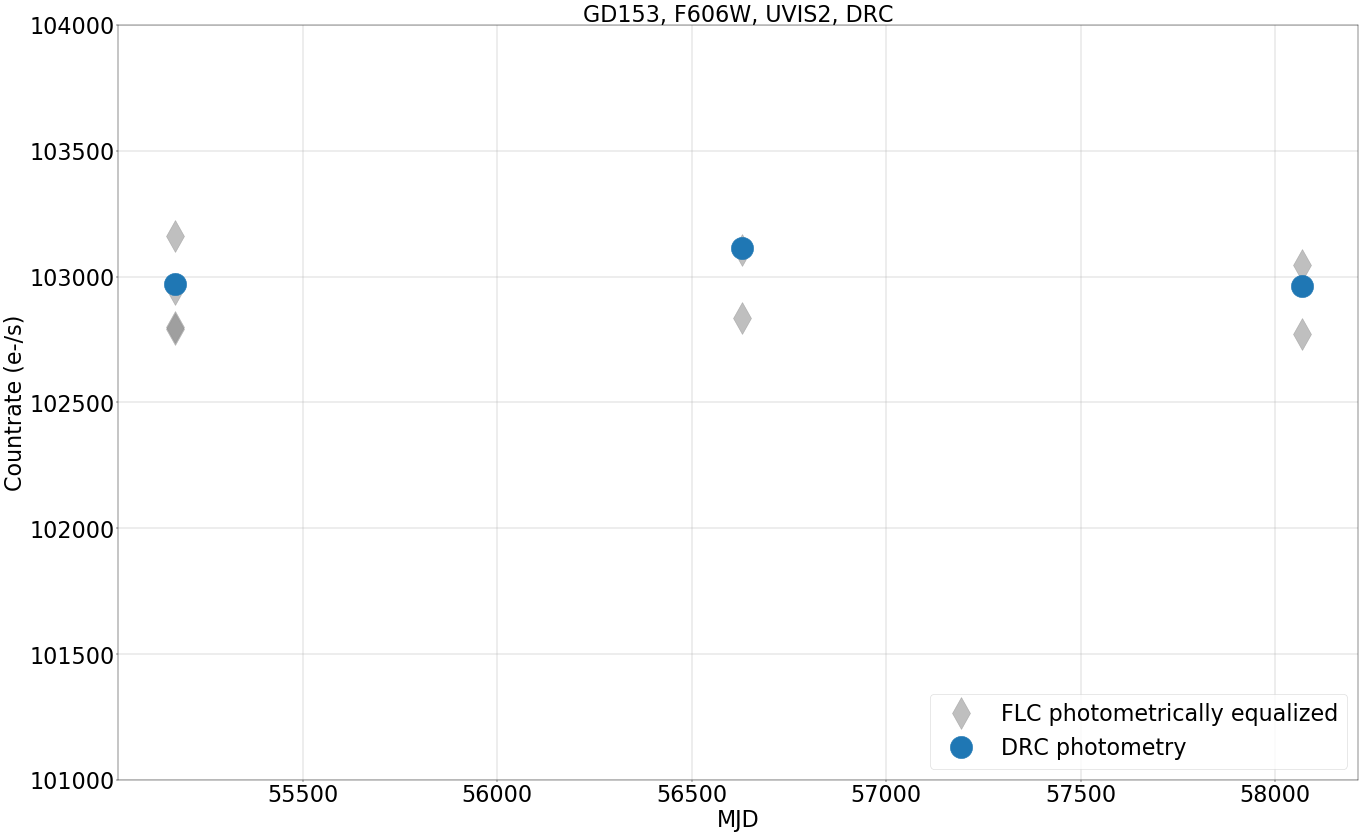In :
fig = plt.figure(figsize=(40, 25), dpi=40)

plt.plot(df['MJD'], df['STMags'], 's', markersize=50, label='FLC', alpha=0.4, color='Grey')
plt.plot(mjds, mags, 'o', markersize=40, label='DRC')
plt.grid()
plt.ticklabel_format(useOffset=False)
plt.xlabel('MJD', fontsize=40)
plt.xticks(fontsize=40)
plt.yticks(fontsize=40)
plt.ylabel('STMAG (Magnitude)', fontsize=40)
plt.ylim(13.55, 13.65)
plt.title('GD153, F606W, UVIS2, DRC', fontsize=40)
plt.legend(loc=4, fontsize=40)

Out:
<matplotlib.legend.Legend at 0x7fdcf0ef4438>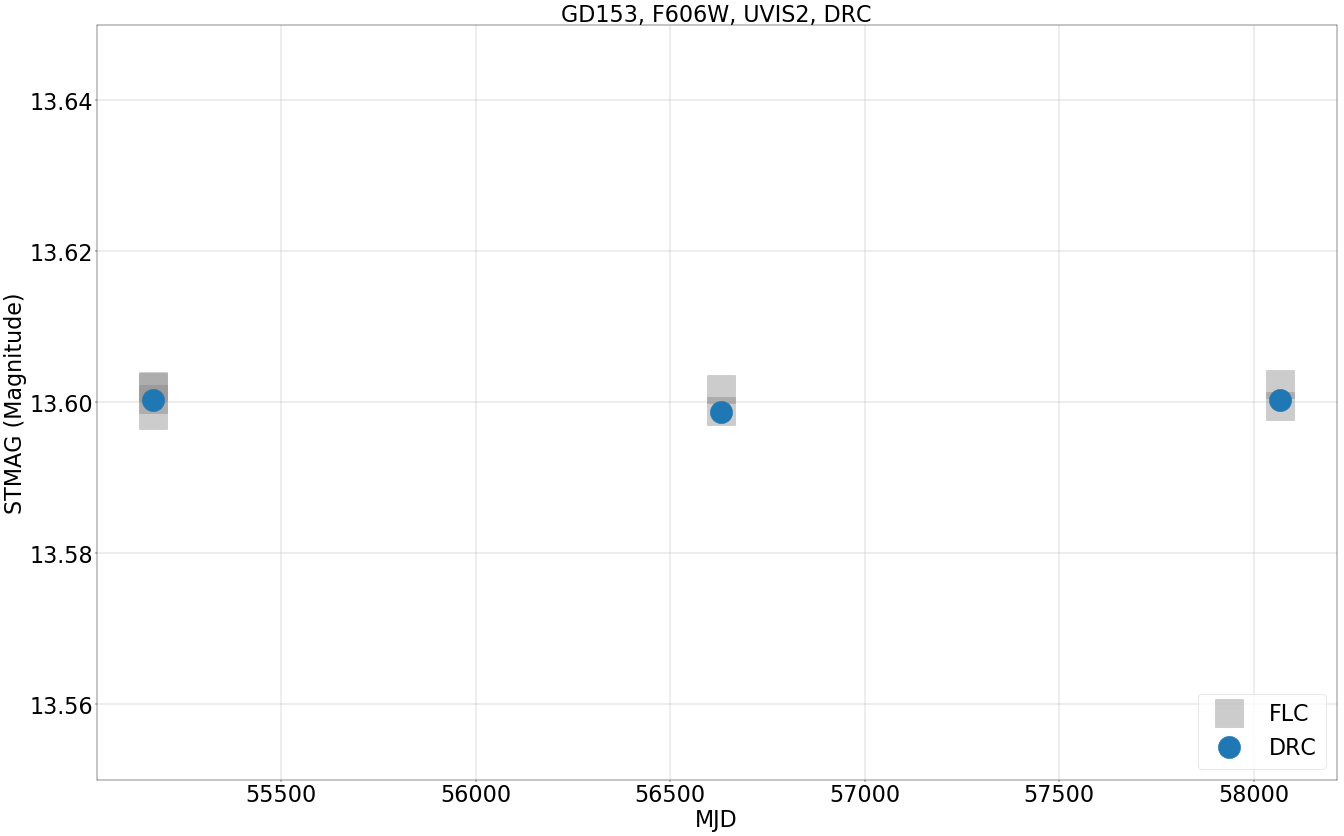In [ ]: